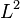# Cross-validation set

A cross-validation set is a set of labeled examples (input-output pairs) used in supervised learning algorithms for the goal of hyperparameter optimization for the learning algorithm. It is distinguished from the training set, on which we run the learning algorithm to determine the parameters. The cross-validation set may be used to tune the values of model hyperparameters (such as the degree of the polynomial to use), regularization hyperparameters (such as the coefficient to use for$L^1$- or$L^2$-regularization), or learning algorithm hyperparameters (such as the learning rate or the number of iterations).